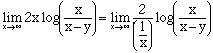Subject: calculus question The limit of the following as x goes to infinity is 2*y. Y is a constant. lim 2*x*log(x/(x-y)) = 2*y I don't understand, though, how this answer is arrived at. Can you help? Thanks, Dan Hi Dan, As x goes to infinity x/(x-y) approaches 1 and hence log(x/(x-y)) approaches log(1) = 0. Thus as x goes to infinite your limit approaches infinity times zero, which makes it a candidate for l'Hospital's Rule. To put the limit into the form of "0/0" writeNow apply l'Hospital's Rule. Cheers, Harley Go to Math Central